Worksheets and No Prep Teaching Resources
Math Worksheets

# Number Bonds Worksheets

Number bonds are a fascinating way for students to make number connections and learn basic math facts! These dynamic worksheets make understanding basic number relationships exciting and will help students develop critical thinking skills at a young age. Explore the various puzzles and worksheets to find the perfect match for each student. Number bonds will help students bond with numbers!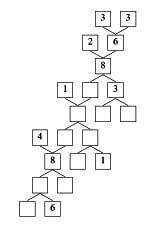Number Bond Puzzles

Tracing - Number Bond Puzzle with Trace Font - Trace the Numbers (all the numbers given)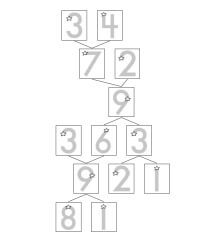Trace the Numbers - Number Bonds Puzzle - Numbers to 9

Tracing - Number Bond Puzzle with Trace Font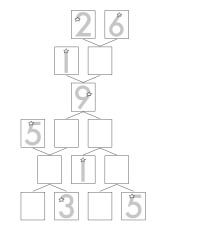Half the Numbers Given - Number Bonds Puzzle - Numbers to 9 One-Fourth the Numbers Given - Number Bonds Puzzle - Numbers to 9 Half the Numbers Given - Number Bonds Puzzle - Numbers to 19 One-Fourth the Numbers Given - Number Bonds Puzzle - Numbers to 19

Number Bond Puzzles: Numbers to 9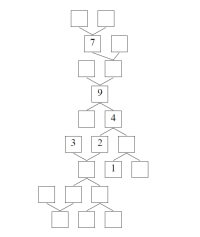Half the Numbers Given - Number Bonds Puzzle - Numbers to 9 One-Fourth the Numbers Given - Number Bonds Puzzle - Numbers to 9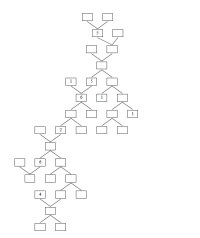Half the Numbers Given - Number Bonds Puzzle - Numbers to 9 (smaller boxes - more per page) One-Fourth the Numbers Given - Number Bonds Puzzle - Numbers to 9 (smaller boxes - more per page)

Number Bond Puzzles: Numbers to 19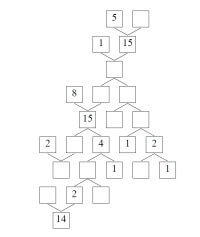Half the Numbers Given - Number Bonds Puzzle - Numbers to 19 One-Fourth the Numbers Given - Number Bonds Puzzle - Numbers to 19

Number Bond Puzzles: Numbers to 29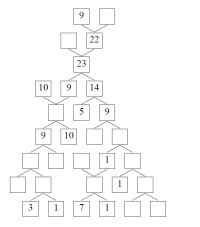Half the Numbers Given - Number Bonds Puzzle - Numbers to 29 One-Fourth the Numbers Given - Number Bonds Puzzle - Numbers to 29

Introduction to Number Bonds: Using Pictures to Complete Number Bonds

Tracing - Black and White Rectangles - Complete the Number Bond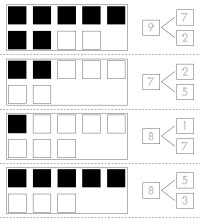Rectangles: All the Answers Given (just trace the numbers) Rectangles: Half the Numbers Given Rectangles: One-Fourth the Numbers Given Rectangles: All Blank

Tracing - Pictures - Complete the Number Bond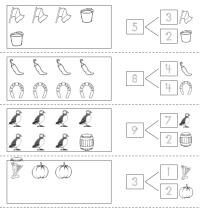All the Answers Given (just trace the numbers) Half the Numbers Given One-Fourth the Numbers Given All Blank

Black and White Rectangles - Complete the Number Bond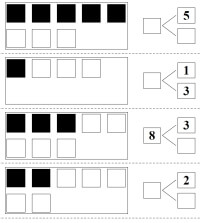Rectangles: Half the Numbers Given Rectangles: One-Fourth the Numbers Given Rectangles: All Blank

Pictures - Complete the Number Bond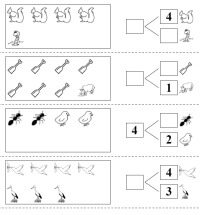Half the Numbers Given One-Fourth the Numbers Given All Blank

Number Bonds

Complete the Number Bonds: Numbers to 9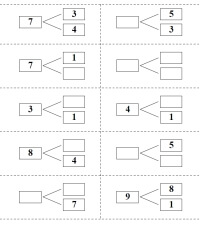Half the Numbers Given One-Fourth the Numbers Given All Blank

Complete the Number Bonds: Numbers to 19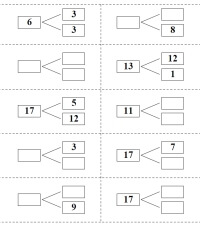Half the Numbers Given One-Fourth the Numbers Given All Blank

Sums: Matching Numbers to get a Sum

Matching Sums Up to 9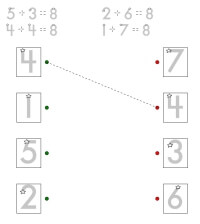Matching Sums Up to 9: 1 answer line given and header gives equations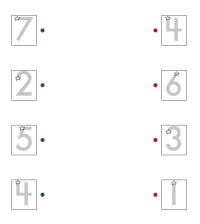Matching Sums Up to 9: 1 answer line given Matching Sums Up to 9

Matching Sums of 10 to 19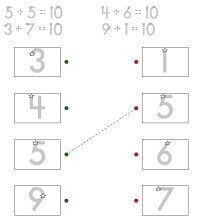Matching Sums of 10 to 19: 1 answer line given and header gives equations Matching Sums of 10 to 19: 1 answer line given and header gives equations (smaller font - more can be shown)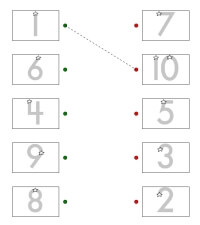Matching Sums of 10 to 19: 1 answer line given Matching Sums of 10 to 19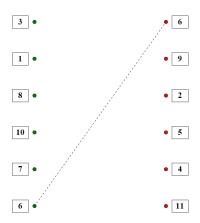Matching Sums of 10 to 19: 1 answer line given (smaller font - more can be shown) Matching Sums of 10 to 19 (smaller font - more can be shown)

Have a suggestion or would like to leave feedback?